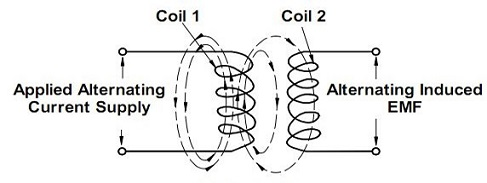# Self and Mutual Inductance

### Self Inductance:

The ability of an inductor to induce voltage in itself when the current changes is called its self inductance or simply inductance. It is denoted by the letter L and its units are Henrys (H).Illustration of Self InductanceSelf Inductance Coil

It can be expressed as, L = VL/(di/dt)

Where VL is the induced voltage in volts
di/dt is the rate of change of current in amperes per second.

If di/dt = 1 A/s and VL = 1v, then L = 1H

Hence a coil has an inductance of one henry if an emf of one volt is induced in it when current through it changes at the rate of 1 A/s.

Physical factors affecting the value of inductance (L):

It is found that whenever current through an inductor changes (i.e. increases or decreases), a counter emf is induced in it which tends to oppose this change. This property of the coil due to which it opposes any change of current through it is called inductance (L).

In terms of physical factors
L = μ0μrAN2/l Henrys

Where μ0 = absolute permeability of air or vacuum.
μr = relative permeability of the core material.
A = cross-sectional area of core
N = number of turns of the coil
l = core length

### Mutual Inductance (M):

The ability of varying current in one coil to induce voltage in a nearby coil is known as the mutual inductance. It is denoted by the letter M or LM and its units are Henrys (H).Illustration of Mutual Inductance

It can be expressed as M= VL2/(di1/dt)

Where VL2 is the mutually-induced emf produced in the second coil (di1/dt) is the rate of change of current through the first coil.

Physical Factors Affecting the Value of Mutual Inductance (M):

When two coils are placed so close to each other that the expanding and collapsing magnetic flux of one coil links with the other, an induced emf is produced in the other coil. These two coils are then said to have mutual inductance (M).

In terms of physical factors,

M = μ0μrAN1N2/l
Where ‘l’ is the length of the magnetic path.

### Coefficient of Coupling (K):

The degree of magnet coupling is known as the coefficient of coupling, denoted by the letter k. If L1 and L2 are the inductances of two inductors then the coefficient of coupling is expressed as
k = Flux linkages between L1 and L2 / Total flux produced by L1
(or)
K = M / (L1L2)

If all the flux due to one coil (L1) links with the other then k = 1. If the flux of one coil (L1) does not at all link with the other coil (L2) then k = 0. Thus k is a constant whose value lies between 0 and 1.

A high value of k = 1, called tight coupling allows the current in one coil to induce more voltage in the other coil. A low value of k called loose coupling induces less voltage. If the coils are kept for apart or kept perpendicular, the coefficient of coupling is zero and hence there is no mutual inductance.

### Types of Inductors:

Inductors are classified in many ways. Some of them are as follows.

1. According to the core material used,
(a) air core inductors
(b) iron core inductors
(c) ferrite core inductors

2. According to the frequency of operation
(a) audio frequency (AF) coils